• 题解：范德蒙恒等式(快速幂+逆元+排列组合) 这个题就是用上面来写，图中的a 就是 L【i】，b就是R【i】，也就是如果从左边再选出X个，右边就要选出X+1个来对应。0(L【i】-1 ， R【i】)；然后把所有的情况加起来取模...

Anton and School - 2
As you probably know, Anton goes to school. One of the school subjects that Anton studies is Bracketology. On the Bracketology lessons students usually learn different sequences that consist of round brackets (characters "(" and ")" (without quotes)).
On the last lesson Anton learned about the regular simple bracket sequences (RSBS). A bracket sequence s of length n is an RSBS if the following conditions are met:

It is not empty (that is n ≠ 0).
The length of the sequence is even.
First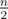charactes of the sequence are equal to "(".
Lastcharactes of the sequence are equal to ")".

For example, the sequence "((()))" is an RSBS but the sequences "((())" and "(()())" are not RSBS.
Elena Ivanovna, Anton's teacher, gave him the following task as a homework. Given a bracket sequence s. Find the number of its distinct subsequences such that they are RSBS. Note that a subsequence of s is a string that can be obtained from s by deleting some of its elements. Two subsequences are considered distinct if distinct sets of positions are deleted.
Because the answer can be very big and Anton's teacher doesn't like big numbers, she asks Anton to find the answer modulo 109 + 7.
Anton thought of this task for a very long time, but he still doesn't know how to solve it. Help Anton to solve this task and write a program that finds the answer for it!
Input
The only line of the input contains a string s — the bracket sequence given in Anton's homework. The string consists only of characters "(" and ")" (without quotes). It's guaranteed that the string is not empty and its length doesn't exceed 200 000.
Output
Output one number — the answer for the task modulo 109 + 7.
Examples

Input
)(()()

Output
6

Input
()()()

Output
7

Input
)))

Output
0

Note
In the first sample the following subsequences are possible:

If we delete characters at the positions 1 and 5 (numbering starts with one), we will get the subsequence "(())".
If we delete characters at the positions 1, 2, 3 and 4, we will get the subsequence "()".
If we delete characters at the positions 1, 2, 4 and 5, we will get the subsequence "()".
If we delete characters at the positions 1, 2, 5 and 6, we will get the subsequence "()".
If we delete characters at the positions 1, 3, 4 and 5, we will get the subsequence "()".
If we delete characters at the positions 1, 3, 5 and 6, we will get the subsequence "()".

The rest of the subsequnces are not RSBS. So we got 6 distinct subsequences that are RSBS, so the answer is 6.
题解：范德蒙恒等式(快速幂+逆元+排列组合)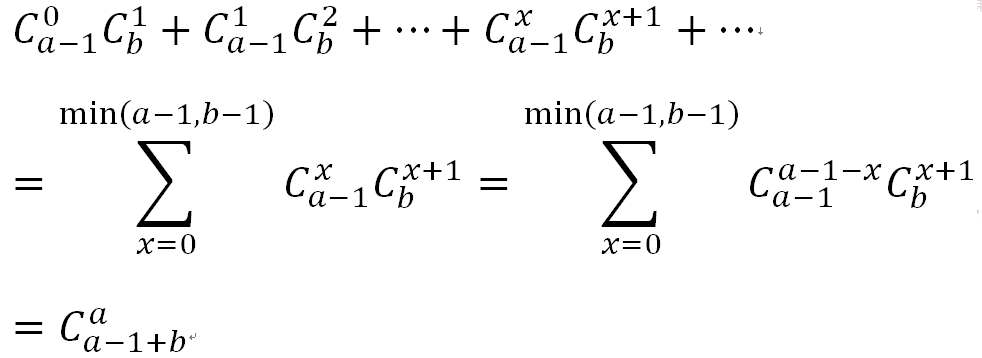这个题就是用上面来写，图中的a 就是 L【i】，b就是R【i】，也就是如果从左边再选出X个，右边就要选出X+1个来对应。0<= X <=min(L【i】-1 ， R【i】)；然后把所有的情况加起来取模，就是答案
写的时候，cin输入，从0开始计算l,r数组，结果出错了，strlen(str)一定要记录下来，不然T到自闭(我太菜了，没想到这个原因)

 1 #include <iostream>
2 #include <algorithm>
3 #include <cstring>
4 #include <string>
5 #include <vector>
6 #include <map>
7 #include <set>
8 #include <list>
9 #include <deque>
10 #include <queue>
11 #include <stack>
12 #include <cstdlib>
13 #include <cstdio>
14 #include <cmath>
15 #include <iomanip>
16 #define ull unsigned long long
17 #define ll long long
18 #define pb push_back
19 #define rep(i,start,end) for(int i=start;i<=end;i++)
20 #define per(i,end,start) for(int i=end;i>=start;i--)
21 #define tle ios::sync_with_stdio(false); cin.tie(0); cout.tie(0);
22 #define lc now<<1
23 #define rc now<<1|1
24 using namespace std;
25 const int mod = 1000000007 ; ///998244353;
26 const int mxn = 2e5 +7;
27 ll _,n,m,t,k,u,v,ans,cnt,ok,lim;
28 int  l[mxn] ,  r[mxn] ;
29 ll dp[mxn] , inv[mxn];
30 char str[mxn];
31 ll ksm(ll x, ll k)
32 {
33     ll ans = 1;
34     while(k)
35     {
36         if(k&1) ans=ans*x%mod;
37         x = x*x%mod;
38         k>>=1;
39     }
40     return ans;
41 }
42 ll C(ll n,ll m){return dp[n]%mod*inv[n-m]%mod*inv[m]%mod;}
43 int main()
44 {
45     tle;
46     dp=1;inv = 1 ;
47     rep(i,1,mxn) dp[i] = dp[i-1]*i%mod , inv[i] = ksm(dp[i] , mod-2);
48     scanf("%s",str+1);
49     int len = strlen(str+1) ;
50     rep(i,1,len) l[i] = ( str[i]=='('?l[i-1]+1:l[i-1] );
51     per(i,len,1) r[i] = ( str[i]==')'?r[i+1]+1:r[i+1] );
52     ll ans = 0 ;
53     for(int i=1;i<=len;i++)
54     {
55         if(str[i]=='(')
56             ans = ( ans + C(l[i]+r[i]-1,l[i]) )%mod ;
57     }
58     printf("%lld\n",(ans%mod));
59 }

Walk
链接：https://ac.nowcoder.com/acm/contest/5929/K来源：牛客网
题目描述

多多喜欢行走，有一天老师问他一个问题：在一个方格点阵中，左上角点的坐标为(1, 1)，行坐标从上到下依次递增，列坐标从左到右依次递增，每次行走可以向上、下、左、右移动一格。现在要从(1, 1)点走到(N, M)点，在行走步数最少的情况下，有多少种行走方法？(答案可能过大，请对答案取模1000000007)
输入描述:
第一行输入一个正整数 T，代表询问次数 (1 ≤ T ≤ 100000)
接下来 T 行，每行输入两个正整数 N，M (1 ≤ N ≤ 106，1 ≤ M ≤ 106)
输出描述:
对于每次询问，输出一个正整数，代表在行走步数最少的情况下，从(1, 1)点走到(N, M)点的方法总数 (答案可能过大，请对答案取模1000000007)

示例1

输入

1
2 2

输出

2

说明

(1, 1)    (1, 2)
(2, 1)    (2, 2)
在步数最少的情况下，从(1, 1)走到(2, 2)的方法有2种：
第一种：(1, 1) -> (1, 2) -> (2, 2)
第二种：(1, 1) -> (2, 1) -> (2, 2)

示例2

输入

1
2 3

输出

3

说明

(1, 1)    (1, 2)    (1, 3)
(2, 1)    (2, 2)    (2, 3)

在步数最少的情况下，从(1, 1)走到(2, 3)的方法有3种：
第一种：(1, 1) -> (1, 2) -> (1, 3) -> (2, 3)
第二种：(1, 1) -> (1, 2) -> (2, 2) -> (2, 3)
第三种：(1, 1) -> (2, 1) -> (2, 2) -> (2, 3)

示例3

输入

1
5 3

输出

15

#include <iostream>
#include <algorithm>
#include <cstring>
#include <string>
#include <vector>
#include <map>
#include <set>
#include <list>
#include <deque>
#include <queue>
#include <stack>
#include <cstdlib>
#include <cstdio>
#include <cmath>
#include <iomanip>
#define pi acos(-1)
#define ull unsigned long long
#define ll long long
#define pb push_back
#define all(vc) vc.begin() , vc.end()
#define rep(i,start,end) for(int i=start;i<=end;i++)
#define per(i,end,start) for(int i=end;i>=start;i--)
#define tle ios::sync_with_stdio(false); cin.tie(0); cout.tie(0);
#define lc now<<1
#define rc now<<1|1
#define eps 1e-9
{
ll x=0,f=1;char ch=getchar();
while(ch<'0'||ch>'9'){if(ch=='-')f=-1;ch=getchar();}
while(ch>='0'&&ch<='9'){x=x*10+ch-'0';ch=getchar();}
return x*f;
}
using namespace std;
const int mod = 1000000007;//998244353;
const int mxn = 2e6 +7;
const int inf = 1000000007;
ll _,n,m,t,k,u,w,v,ans,cnt,ok,lim,len,tmp,last;
struct node{int x,y,t;};//e[mxn];
ll num[mxn];
ll ksm(ll a,ll b)
{
ll ans = 1;
while(b)
{
if(b&1) ans = ans*a%mod;
a=a*a%mod;
b>>=1;
}
return ans;
}
ll C(ll n,ll m)
{return num[n] * ksm(num[m], mod - 2) % mod * ksm(num[n - m], mod - 2) % mod;}
int main()
{
num = 1 ; num = 1 ;
rep(i,2,mxn) num[i] = i*num[i-1]%mod;
cin>>t;
while(t--)
{
cin>>n>>m;
n-- ; m-- ;
cout<<C(n+m,m)<<endl;
}
}

View Code


展开全文lamp 算法 字符串 动态规划
• 今天我们来认识组合数学中一个重要的恒等式---范德蒙恒等式。这个恒等式的表述如下       很自然的公式，接下来一起来看看它的证明，在维基百科上给出了两种方法证明，分别如下   （1）组合方法证明   ...
今天我们来认识组合数学中一个重要的恒等式---范德蒙恒等式。这个恒等式的表述如下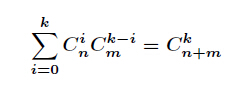很自然的公式，接下来一起来看看它的证明，在维基百科上给出了两种方法证明，分别如下

（1）组合方法证明

甲班有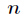个同学，乙班有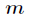个同学，从两个班中选出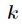个一共有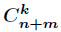种不同的选法。而换一种思维方式

从甲班中选取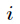个同学，从乙班中选取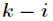个同学，共有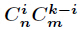种方法，而对所有的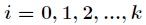就是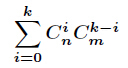可以看出这两种方法应该是相等的，即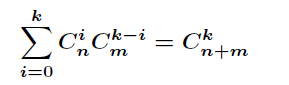（2）生成函数法证明

由于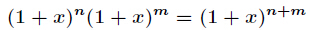，对于等式左边有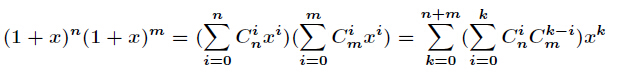而对于等式右边有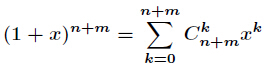左右两边一比较可知成立，证明完毕！

接下来我们看看一些关于范德蒙恒等式的衍生问题。

（1）证明下面恒等式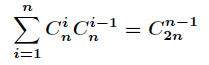证明：由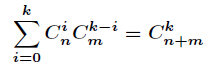令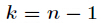和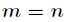，那么有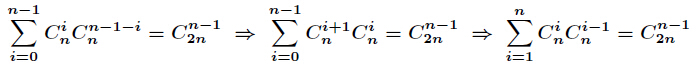证明完毕！

（2）证明下列的恒等式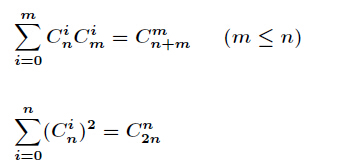展开全文组合
• 关于峁诗松1－2习题第五题，困扰了自己两天，终于通过《组合理论及其应用》4－1中找到答案，才了解到此题其实是范德蒙恒等式。 其实书中给出的解答，对于没接触过的人来说，是无法理解的，更好的解释是维基百科上...
关于峁诗松1－2习题第五题，困扰了自己两天，终于通过《组合理论及其应用》4－1中找到答案，才了解到此题其实是范德蒙恒等式。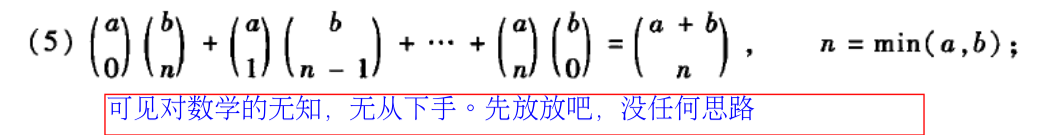其实书中给出的解答，对于没接触过的人来说，是无法理解的，更好的解释是维基百科上。如下：

组合证明法：

甲班有个同学，乙班有个同学，从两个班中选出个一共有种不同的选法。而换一种思维方式， 从甲班中选取个同学，从乙班中选取个同学，共有种方法，而对所有的，就是可以看出这两种方法应该是相等的，即对于生成函数法的证明，只要了解一下就可以，详细的话就要研究《组合理论及其应用》4-1章节

由于对于等式左边有：而对于等式右边有左右两边一比较可知展开全文• 范德蒙恒等式，听起来牛逼哄哄，但是并没有那么晦涩深奥 其表述如下 $\sum_{i=0}^{k}\dbinom{n}{i}\dbinom{m}{k-i}=\dbinom{n+m}{k}$ 组合方法证明： 考虑这样一个问题，甲班有$n$个同学，乙班有$m$个同学，从两...
范德蒙恒等式，听起来牛逼哄哄，但是并没有那么晦涩深奥
其表述如下
$\sum_{i=0}^{k}\dbinom{n}{i}\dbinom{m}{k-i}=\dbinom{n+m}{k}$
组合方法证明：
考虑这样一个问题，甲班有$n$个同学，乙班有$m$个同学，从两班选出$k$个人一共有$\dbinom{n+m}{k}$种方式
当然我们也可以枚举甲班选了几个人，即$\sum_{i=0}^{k}\dbinom{n}{i}\dbinom{m}{k-i}$
证毕
生成函数法证明:
$(1+x)^n(1+x)^m=(1+x)^{n+m}$
对于等式左边$(1+x)^n(1+x)^m=(\sum_{i=1}^{n}\dbinom{n}{i}x^i)(\dbinom{m}{i}x^i)=\sum_{k=0}^{n+m}(\sum_{i=0}^{k}\dbinom{n}{i}\dbinom{m}{k-i})x^k$
对于等式右边$(1+m)^{n+m}=\sum_{i=0}^{n+m}\dbinom{n+m}{i}x^i$
上下比较一下，得证
范德蒙恒等式的衍生问题
(1)$\sum_{i=1}^{n}\dbinom{n}{i}\dbinom{n}{i-1}=\dbinom{2n}{n-1}$
有范德蒙恒等式可得$\sum_{i=0}^{k}\dbinom{n}{i}\dbinom{m}{k-i}=\dbinom{n+m}{k}$
令$k=n-1,m=n$
$\sum_{i=0}^{n-1}\dbinom{n}{i}\dbinom{n}{n-1-i}=\sum_{i=0}^{n-1}\dbinom{n}{i}\dbinom{n}{i+1}=\sum_{i=1}^{n}\dbinom{n}{i-1}\dbinom{n}{i}$
$=\dbinom{2n}{n-1}$
证毕
(2)$\sum_{i=0}^{n}\dbinom{n}{i}^2=\dbinom{2n}{n}$
左式$=\sum_{i=0}^{n}\dbinom{n}{i}\dbinom{n}{n-i}=\dbinom{2n}{n}$

转载于:https://www.cnblogs.com/xxzh/p/10655564.html
展开全文• 那么方案数就应该是下面的 1 ， 然后不断化简， 通过范德蒙恒等式 ， 可以将其化为一个组合数。 代码： #include<bits/stdc++.h> using namespace std; #define Fopen freopen("_in.txt","r",...
• 先说要用到的两个公式： Cnm=Cnm−1+Cn−1m−1C_{m}^{n} = C_{m-1}^{n} + C_{m-1}^{...范德蒙恒等式 Ckm+n=∑i=0kCimCk−inC_{m+n}^{k} = \sum_{i=0}^{k}C_{m}^{i}C_{n}^{k-i} 这道题的话很容易发现要求的是: 对于每一codeforces
• Codeforces Round #404 (Div. 2) D. Anton and School - 2 [范德蒙恒等式]codeforces
• http://codeforces.com/problemset/problem/785/D 题目大意： 长度为偶数 左侧全为 '(' 右侧全为 ')' ...首先 范德蒙恒等式 枚举分界线 每一个分界线记录其左侧 '(' 数量和右侧 ')' 数量（包含本组合数
• 搜了题解发现用到了一个叫范德蒙恒等式的东西。 具体思路就是，先统计出所有的右括号。然后从左遍历，每遇到右括号，右括号数量减一。每遇到一个左括号，就可以和之前的左括号组合成1个，2个，3个。。。左括号，再...
• 范德蒙恒等式： 题目大意：给你一串字符串（只包含字符'('和')'），求有多少个子串满足：长度是偶数，且左半边只有'(' 右半边只有')'，比如"((()))"是一个满足条件的字符串。 解题思路：先...
• 代码中的子是C(L[i]-1+R[i],R[i]-1) 因为题解中的那个L[i]代表的是i位置左边有多少个'('. 而代码中的L[i]包括的i个位置。 代码： #include using namespace std; typedef long long ll; const int maxn=2...
• Codeforces 785D-Anton and School - 2 题目原址 [ ...] ...给一组只含 ’ ( ’ 和 ’ ) ’ 的字符串，你可以 ...同时满足 1....删除不同位置括号得到好括号组算作...//范德蒙恒等式 printf ( "%I64d\n" , ans ) ; }Codeforces
• 题目链接：Codeforces-785D-Anton and School - 2每当扫描到一个左括号时，设这个左括号必选，左边有 aa 个左括号（包括其本身），右边有 bb 个右括号，那么有 ∑min(a−1,b−1)i=0Cia−1Ci+1b...根据范德蒙恒等式Cka+b
• 题意：给你一个由'('和')'组成的字符串，问你有多少个子串，前半部分是由'('组成后半部分由')'组成 ...根据范德蒙恒等式计算得出 代码： #include <bits/stdc++.h> #define ll long l...
• 前缀的后缀、 范德蒙恒等式、容斥 子串为 前缀的后缀，这里是子序列，所以s[i]为必取，作为序列的最后一个元素，然后前面的"("为选取， 所以可以预处理出suml和sumr，分表表示i的左边有多少个"(", i的右边有多少个")...Codeforces 子序列
• 那么对于右边的式子：我们再考录x的k次方，即可得到范德蒙恒等式。 那么对于我们的上边的式子可以做出一下化简：   这样对于每一个点，我们在预处理之后，就可以O(1)的计算这个点对答案的贡献。代码如下：...
• As you probably know, Anton goes to school. One of the school subjects that Anton studies is Bracketology. On the Bracketology lessons students usually learn different sequences that consist of...
• 范德蒙恒等式： 经过合理的转化，可以化成C(l+r-1,l)；（推导过程就不啰嗦了） 【代码】： [cpp]   view plain   copy #include   ...
• 做法：自己想了很久没点思路，搜的题解，是“范德蒙恒等式”，第一次见。学习来自： https://blog.csdn.net/zengaming/article/details/63684635 先记录每个字符左边有多少个'('，右边有多少个')'，包含当前字符...
• 遇到右括号,记录, 遇到左括号 组合计算, 应用范德蒙式化简: 在 利用乘法逆元 计算组合数 : AC代码: #include #include #include #include #include #include #include #include #include #...codeforces
• 范德蒙恒等式   #include #include #include #include #include #include #include #include #include #include #include using namespace std; typedef long long ll; const int inf = 0x3f3f3f3f...
• 题目链接 题意：  问你一共有多少个子串，满足下列条件：  ①长度为偶数  ...而由范德蒙不等式可得结果。 PS：求组合数时可以先将所有阶乘都预处理出来，在直接求即可 代码如下： #include #inclucodeforces 数学...